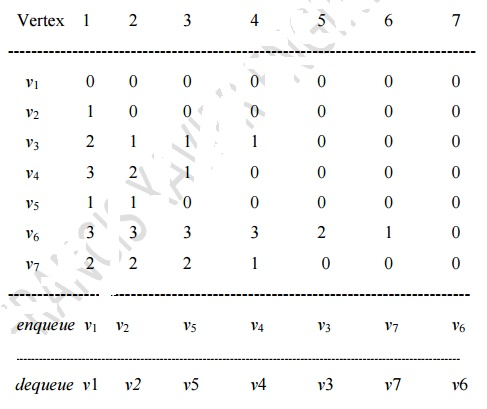Home | | Programming and Data Structure II | Topological Sort

# Topological Sort

A topological sort is an ordering of vertices in a directed acyclic graph, such that if there is a path from vi to vj, then vj appears after vi in the linear ordering.

TOPOLOGICAL SORT:

A topological sort is an ordering of vertices in a directed acyclic graph, such that if there is a path from vi to vj, then vj appears after vi in the linear ordering.

Topological ordering is not possible if the graph has a cycle, since for two vertices v and w on the cycle, v precedes w and w precedes v.

Steps for implementing the topological sort Step 1: Find the indegree for every vertex.

Step 2: Place te vertices whose indegree is 0 on the empty queue.

Step 3: Dequeue the vertex V and decrement the indegreeÔÇčs of all its adjacent vertices.

Step 4: Enqueue the vertex on the queue, if its degree falls to zero.

Step 5: Repeat from step 3 until the queue becomes empty.

Step 6: The topological ordering is the order in which the vertices dequeued.

Routine for Topological Sort

void Topsort( Graph G )

{

Queue Q;

int Counter = 0; Vertex V, W;

Q = CreateQueue( NumVertex );

MakeEmpty( Q );

for each vertex V

if( Indegree[V] = = 0 ) Enqueue( V, Q );

while( !IsEmpty( Q ) )

{

V = Dequeue( Q );

TopNum[V] = ++Counter; /* assign next number */ for each W adjacent to V

if( --Indegree[W] = 0 ) Enqueue( W, Q );

}

/                            if( Counter != NumVertex )

Error("Graph has a cycle");

DisposeQueue( Q ); /* free the memory */

}

Example: Find the topological sort for the following graph.Solution

1.  Number of 1ÔÇ×s present in each column of adjacency matrix represents the indegree of

the corresponding vertex.

Indegree [V1] = 0           Indegree [V5] = 1

Indegree [V2] = 1           Indegree [V6] = 3

Indegree [V3] = 2           Indegree [V7] = 2

Indegree [V4] = 3

2.     Enqueue the vertex, whose indegree is 0 and place it on the queue. Indegree of V1 is 0. So place it in the queue.

3.     Dequeue the vertex V1 from the queue and decrement the indegrees of its adjacent vertex V2 & V3. Indegree [V2] = 0, Indegree [V3] = 1. Now enqueue vertex V2 because its indegree is 0.

4.     Dequeue the vertex V2 from the queue and decrement the indegrees of its adjacent vertex V2 & V3. Indegree [V2] = 0, Indegree [V3] = 1. Now enqueue vertex V2 because its indegree is 0.Algorithm Analysis

The running time of this algorithm is O(|E| + |V|) where E represents the Edges and v represents the vertices of the graph.

Study Material, Lecturing Notes, Assignment, Reference, Wiki description explanation, brief detail
Programming and Data structures : Graphs : Topological Sort |